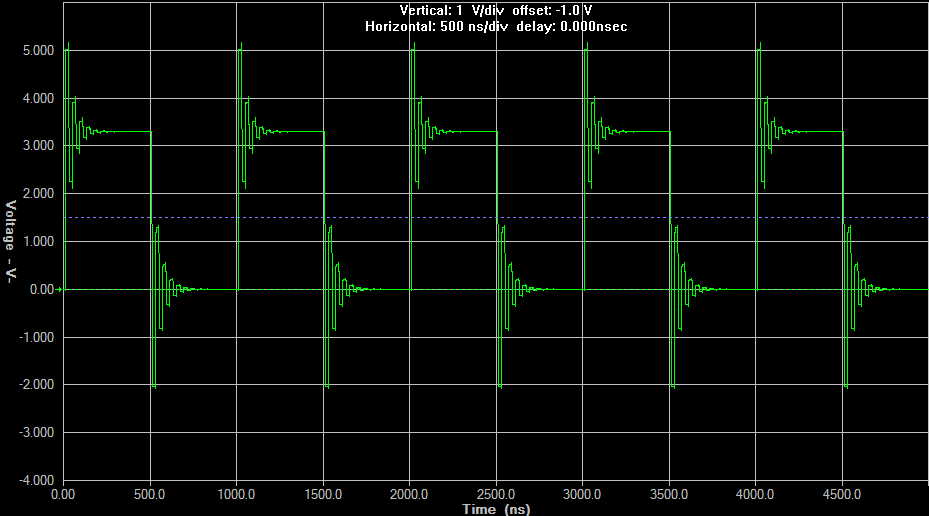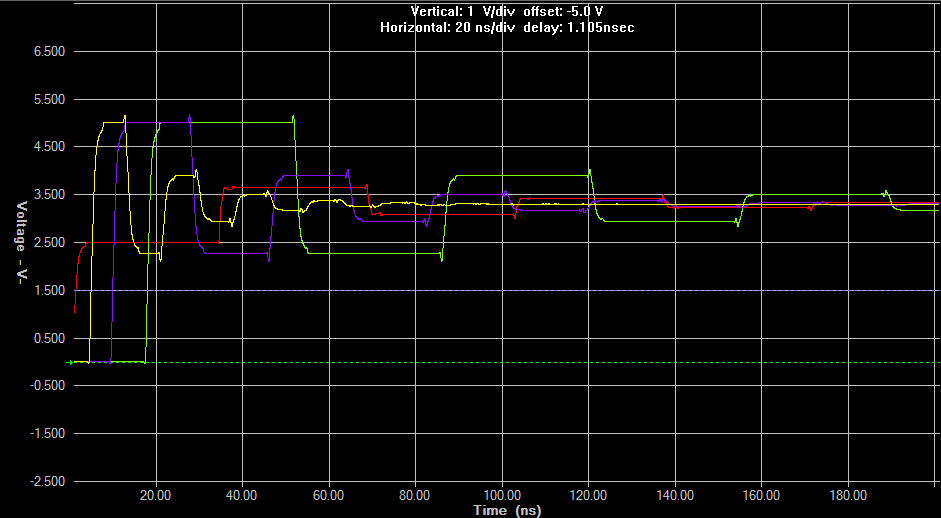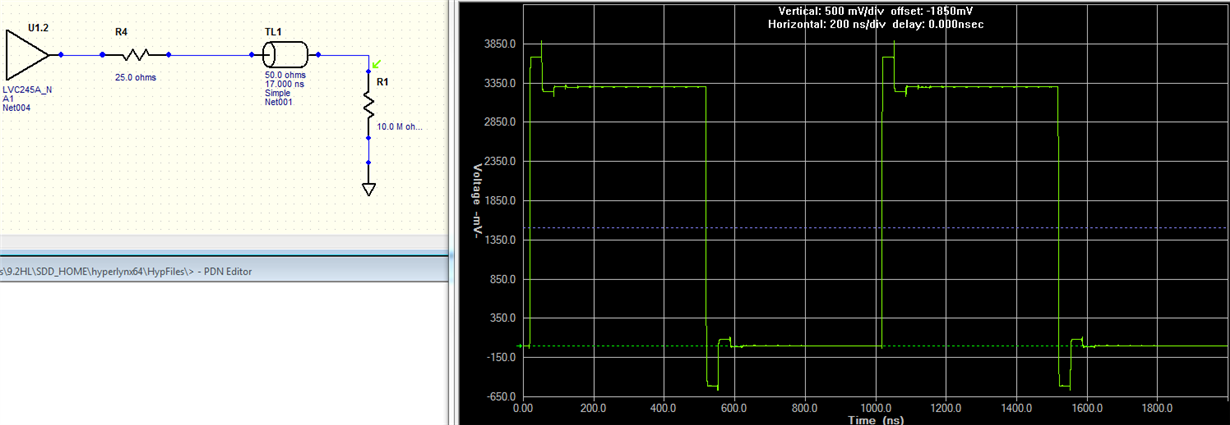If you have a related question, please click the "Ask a related question" button in the top right corner. The newly created question will be automatically linked to this question.

# [FAQ] What happens when I connect a logic device's output to a 50 ohm transmission line?

FAQ: Logic and Voltage Translation > Output Parameters >> Current FAQ

Transmission lines cause a lot of confusion for engineers. I hope to clear up some of that here. I will break this up into a few sections, so if you already know something you can easily skip to the information you need to know.

** Just as a disclaimer, transmission lines are an extremely complex topic, and I could never cover everything related to them here. **

## What is a transmission line?

For the purposes of this post, a transmission line is just a wire that has been designed to carry an electrical signal with minimal loss over relatively large distances. We typically see these in a few forms:

Stripline/microstrip: these are traces on a PCB. Any trace can become a transmission line if it gets long enough. These are the most common source of issues for system designers if transmission lines effects are not taken into account.

Coax cables: These are typically 50-Ω characteristic impedance cables designed specifically for matching RF signals to 50-Ω signal sources and 50-Ω loads. There are other impedances out there, however 50 Ω is the most common version. The transmission line consists of center conductor surrounded by an insulator, and then a tube of conductive material. The signal typically rides on the center conductor and the outer conductor is grounded.

Twisted Pair: These are exactly what they sound like - a pair of wires, usually with one signal and one ground, that are twisted together to form a double  helix. They are most commonly found in network cables like Cat5e. Network cables have a typical characteristic impedance of 100 Ω.

Parallel Line: These are any two wires in parallel, one with the signal and the other grounded (typically). The most common form of these is a ribbon cable, which just has a bunch of wires in parallel running from one location to another. They are not used as commonly today due to noise and interference concerns.

## How long does a transmission line need to be before it starts acting like a transmission line?

Generally speaking for logic? 20 cm

This value is heavily related to the frequency content of a signal, so the faster your edges are, the smaller that number gets. Let's quickly cover what I mean by "frequency content."

### Frequency content of a square wave

Any signal can be broken down into a series of sine waves, and square waves end up being an infinite series of sine waves summed together. There's a great explanation here if you're interested: https://en.wikipedia.org/wiki/Square_wave

So, in a real signal, the maximum edge rate is the primary control on the frequency content of the signal. Let's use a 1 MHz signal as an example.  Here's 3 square waves that are all 1 MHz signals:Three 1 MHz signals, each with a different edge rate. Top to bottom: 100ns, 10ns, 1ns

I took the fourier transform of these, and found that the harmonics for the first signal had already dropped below 0.5V after just 20 MHz, while the third signal still had a 0.518V component at 100 MHz.

The general rule of thumb for determining the bandwidth of a square wave is to use the transition time (t_t, 10% to 90%) of the signal in this equation:

BW = 0.35 / t_t

For the top waveform above, the bandwidth is expected to be 0.35 / 100ns = 3.5 MHz, while the last signal would be 0.35 / 1ns = 350 MHz.

This bandwidth frequency, and not the square wave frequency, is what we are referring to when we talk about the "frequency content" of a signal.

Typical logic signals have transition times between 1ns and 10ns, which puts the typical maximum bandwidth of a signal around 350 MHz.

The general rule of thumb for a transmission line to be considered 'long enough' to start acting like a transmission line is one quarter wavelength ( λ/4 ). To get wavelength we can use this equation for a quick approximation:

λ = c / BW = 300 Mm/s / 350 MHz = 0.857 m

λ/4 ~= 21.4 cm

In the world of under 350 MHz square-wave clock signals (which is where standard logic devices 'live'), a "long distance" is at least 21.4 cm. On many circuit boards and in many systems, 20 cm isn't that large of a distance, so you might start to see transmission line effects on your board.

It is possible to start seeing transmission line effects at as low as one tenth wavelength ( λ/10 ), which would mean 8.6 cm for our typical logic circuit.

## What exactly does a 'characteristic impedance' mean?

The characteristic impedance of a transmission line is simply the ratio of voltage to current at any point in the transmission line. The proof of this is complex, so I won't explain here, however just know that when you see "50 Ω" on a transmission line, it DOES NOT mean that the line acts like a 50 Ω resistor. It means that the travelling waves on that line will have a ratio of voltage to current that is 50 Ω, after all, resistance as defined by Ohm's Law is just a ratio:

R = V / I

This has some very interesting implications, which will be covered in the next section.

## What are these 'transmission line effects' that keep coming up?

When a signal travels over a transmission line, there is typically very little loss (which is why we use transmission lines), however it takes time for the signal to propagate through the line. This time component is the primary source of issues.

### Signals can reflect on a transmission line if there is an impedance mismatch.

From the previous section, we know that at -any point- on a transmission line, the voltage to current ratio will always be the same value (given as Z_0, aka the characteristic impedance of the transmission line).

What happens when you connect a resistor to the end of the transmission line, forcing it to have a different voltage to current ratio (say 50 Ω on the transmission line and a 100 Ω resistor at the load)?

When the ratio of voltage to current is different on the transmission line and at the load, this produces a reflected wave.

Here's 3 more square waves measured at the load, this time having different load terminations with everything else being held constant:100 Ω termination, 50 Ω transmission line1 kΩ termination, 50 Ω transmission line10 MΩ termination, 50 Ω transmission line (most typical impedance for a CMOS input)

You can see in the above images that the overshoot and oscillation on the line gets worse as the termination impedance gets larger. This is because the impedance match to the transmission line is getting worse. Note also that the input waveform was a 3.3-V square wave, and the output is reaching over 5 V in the last example. The reflections on the line are additive, resulting in large voltages that can be damaging to CMOS devices.

### Signals are delayed by a transmission line

The output signal will have a delay based on the length of a transmission line. Here's an example with 3 different length lines, showing the input (red) and outputs (yellow, purple, green) in the same plot:* Only the input for the green line is shown (in red), the other inputs start at the same time.

This image shows a few interesting things.

First, the input is not a clean square wave due to the reflections on the line.

Next, the delays are noticeably different for the initial rising edge of the signal.

Finally, the oscillation (aka ringing) on the signals is related to the delay. You can see that the green signal oscillated much longer than the yellow signal due to the longer delay on the line -- this is because reflections take longer to go back and forth on the line.

## What can I do to keep transmission lines from affecting my logic signals?

Some people think the best solution is to impedance match the signals. Although this will eliminate reflections, it will introduce new problems.  Here's a (close to) perfectly matched impedance signal output waveform:Output of 3.3V signal with matched 50 Ω input, Z_0 = 50 Ω, and 50 Ω load.

The signal looks great, however you should note that the output only reaches about half of the input voltage, which is what we would expect (50 Ω input from source, 50 Ω load... Vout = Vcc * 50/(50 + 50) = V_CC/2). This is generally a bad thing in logic circuits.

The best options that I know of is to use a damping resistor at the input to match the input as much as possible to the transmission line, as shown here:The circuit on the left shows an added damping resistor of 25 Ω. The LVC series of logic has output drive strength close to 25 Ω, so this added resistor gets us close to the required 50 Ω.

You can see in the output waveform that the ringing is significantly reduced, even though the load is still the same 10 MΩ that was used up in my first example. This signal would not cause any problems when going into a CMOS input.

This method works by eliminating the reflections on their first bounce. There is still a reflection from the high-impedance load, however the signal source is close to a perfect match, so those reflections will not bounce back and forth on the transmission line.